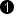## Operator ShiftRight

The operator performs an arithmetic shift of the input data to the right. The number of bits to be shifted is defined using parameter Shift. Each bit at the input is right shifted by the parameterized number of bits.

Bit shifting is usually used to scale a value by a power of two value. For example a right shift by one bit will divide the input value by 2. A right shift by 2 bits will divide the input value by 4.

Each color component is shifted separately.

### I/O Properties

Property Value
Operator Type O
Input Link I, data input
Output Link O, data output

### Supported Link Format

Bit Width [1, 64] unsigned, [2, 64] signed autoArithmetic {unsigned, signed} as I
Parallelism any as I
Kernel Columns any as I
Kernel Rows any as I
Img Protocol {VALT_IMAGE2D, VALT_LINE1D, VALT_PIXEL0D} as I
Color Format any as I
Color Flavor any as I
Max. Img Width any as I
Max. Img Height any as I
 The bit width at the output is the input bit width minus the value of parameter Shift.

### Parameters

Shift
Type static parameter
Default 0
Range

[0, Input BitWidth - 1] for unsigned

[0, Input BitWidth - 2] for signed

This parameter defines the number of bits by which the input is right shifted.

### Examples of Use

The use of operator ShiftRight is shown in the following examples: